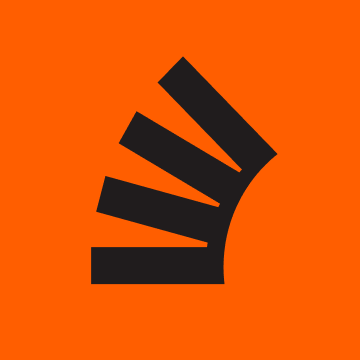# ios – Swapping materials on a ModelEntity in RealityKit

0
28I have a 3D Model (USDZ) in RealityKit that has 1 PBR material. I can select the model via UILongPressGestureRecognizer. When I select it I need the 3D Model to change colour, so the user knows that is been selected. I have kind of got it to work by appending a ‘UnlitMaterial’ which is Orange to the model, then assigning it to material and the PBR to material. Once the 3D Model has been selected, the user unselects the model by ‘LongPress’ again and the 2 materials are swapped and the last is deleted. This works ok first time round, if I start the process again (2 time round) the ‘UnlitMaterial’ is White. On the 3rd time round its Orange?

I tried to placing the ‘var simpleMat = UnlitMaterial(color: .orange)’ at different positions in the ‘If Statements’, because I think when the unlit is white its somehow not correctly assigning the orange colour, its just a Unlitmaterial’ with no colour.

``````@objc func handleLongPress(recognizer: UILongPressGestureRecognizer)  {

let location = recognizer.location(in: arView)

if let entity = arView.entity(at: location){

if let anchorEntity = entity.anchor{

currectAnchorEntity = anchorEntity

switch recognizer.state {
case .began:

//if the model/object is not currently selected and nothing assigned to currentModelEntity (when you first start AR session)
if currentModelEntity != entity && currentModelEntity.children.isEmpty {

var simpleMat = UnlitMaterial(color: .orange)

//---   1   making it the current
currentModelEntity = entity

//---   2  finding the model
entModel = (entity.findEntity(named: "model") as? ModelEntity)!

//---   3  Adding the unlitmaterial colour materal to material 
entModel.model?.materials.append(simpleMat)

//--    4  Assigning Varibles to  &  for reference
let matLastL = entModel.model?.materials.last
let matLastF = entModel.model?.materials.first

//---   5  Swaps the materials on the model, so i can keep the original material
entModel.model?.materials = matLastF!
entModel.model?.materials = simpleMat

recognizer.state = .cancelled

}

// not the current model but there is another model that is selcted which is the 'CurrentModelEntity'
else if currentModelEntity != entity && currentModelEntity.children.count == 1 {

//---   1  finding the model on currently selected model
entModel = (currentModelEntity.findEntity(named: "model") as? ModelEntity)!

//---   2  Assigning Varibles to  &  for reference
let matLastL = entModel.model?.materials.last
let matLastF = entModel.model?.materials.first

//---   3  Assigning the last material(original) to  slot
entModel.model?.materials = matLastL!

//---   4  Removing the 'UnlitMaterial' from 
entModel.model?.materials.remove(at: 1)

//---   5 Unassigning the currentModel
currentModelEntity = Entity()

//---   7  stopping the touches
recognizer.state = .cancelled

}

// is the 'CurrentModelEntity'- at this point the materials are Unlit=, Original=
else if currentModelEntity == entity  && currentModelEntity.children.count == 1{

//---    1  finding the model on currently selected model
entModel = (entity.findEntity(named: "model") as? ModelEntity)!

//---    2  Assigning Varibles to  &  for reference
let matLastL = entModel.model?.materials.last
let matLastF = entModel.model?.materials.first

//---    3  Swaps the materials on the model
entModel.model?.materials = matLastF!
entModel.model?.materials = matLastL!

//---    4  Remove the simpleMat from slot 1
entModel.model?.materials.remove(at: 1)

//---    6  Unasigning the current model
currentModelEntity = Entity()

//---    7  stopping the touches
recognizer.state = .cancelled

}

case .ended, .cancelled: break

default:
break

}

}

}

}
``````